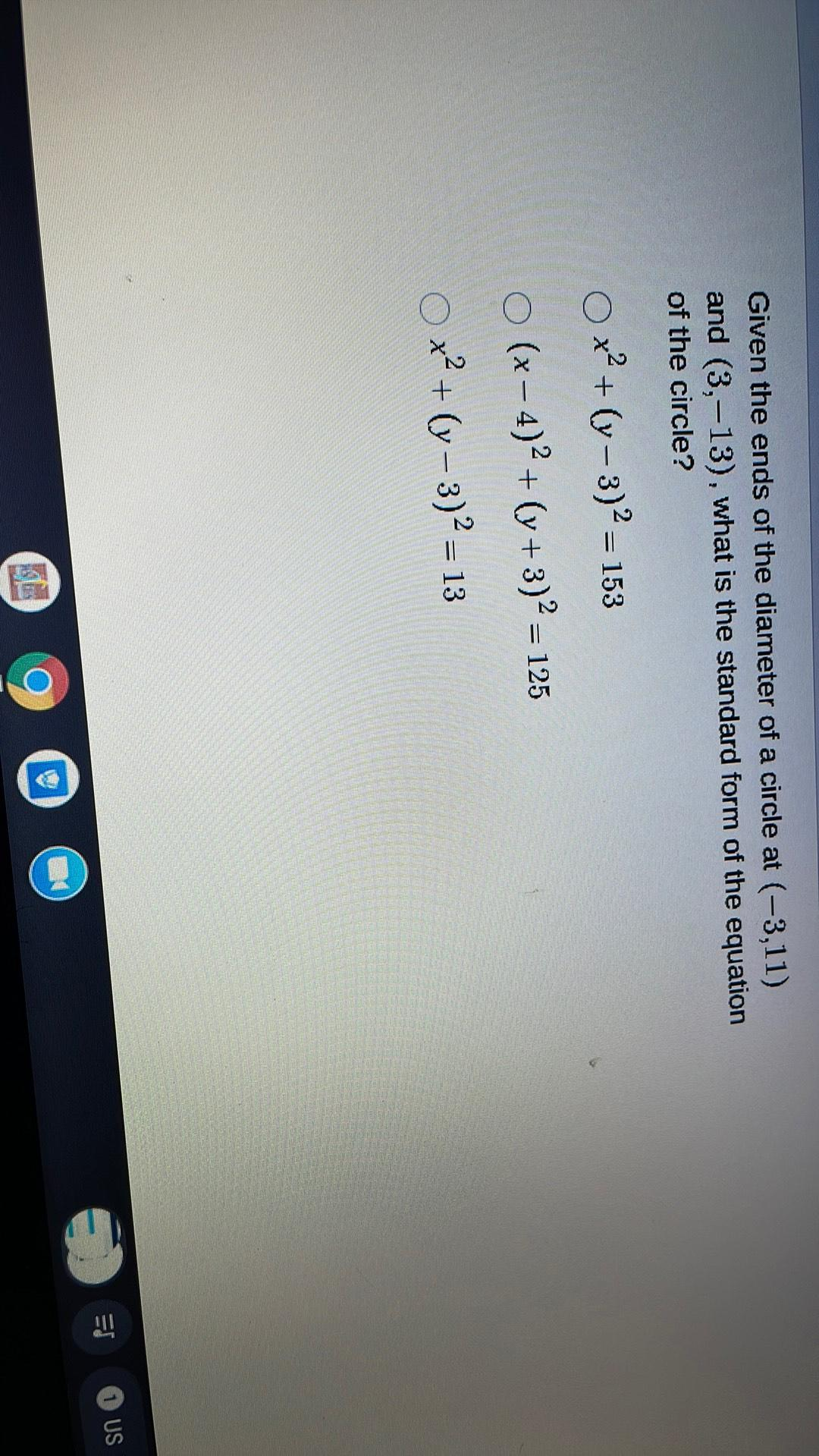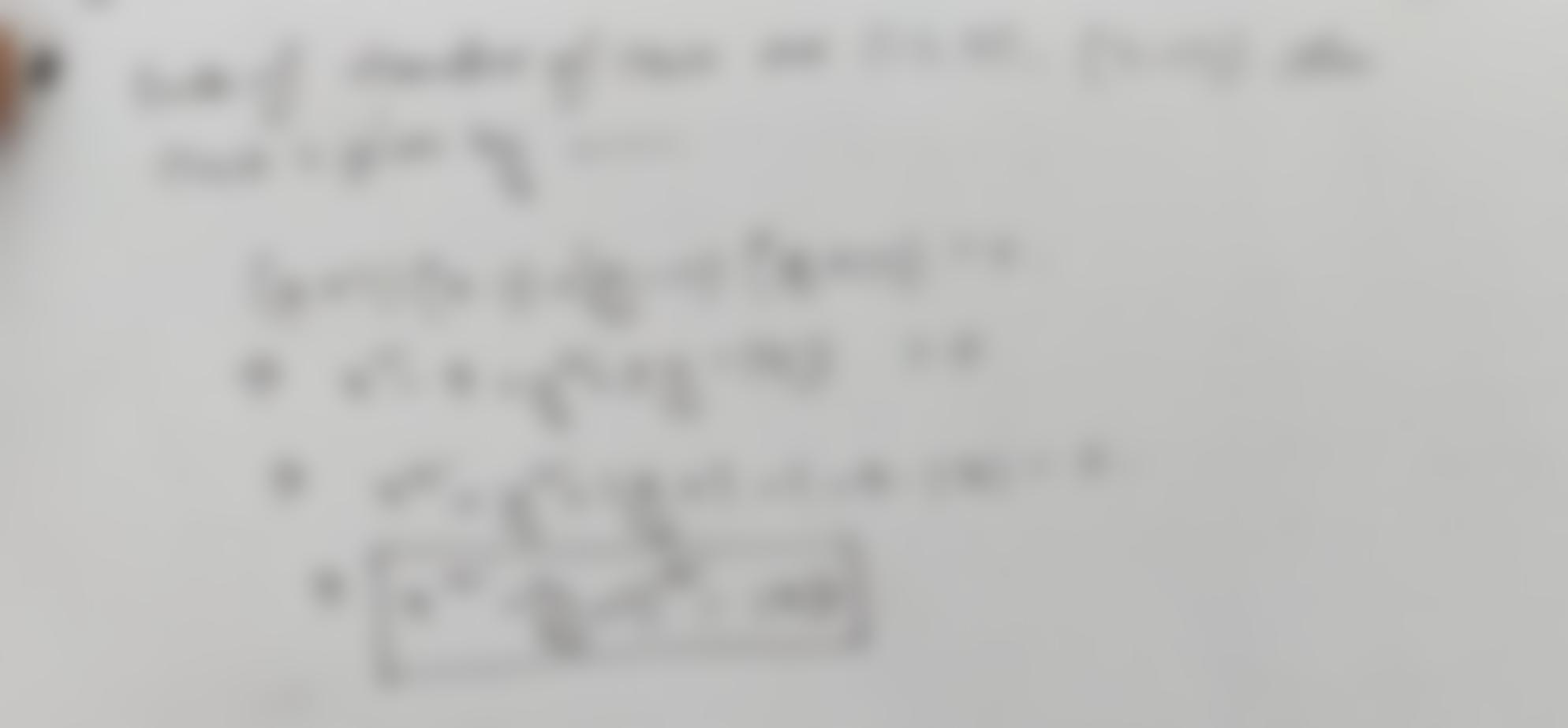Question:

Given the ends of the diameter of a circle at (-3,11) and (3,-13), what is the standard form of the equation of the circle? 1 =Given the ends of the diameter of a circle at (-3,11) and (3,-13), what is the standard form of the equation of the circle? 1 = = O x2 + (y - 3)2 = 153 o (x - 4)2 + (y + 3)2 = 125 o x2 + (y - 3)2 = 13 + ES US